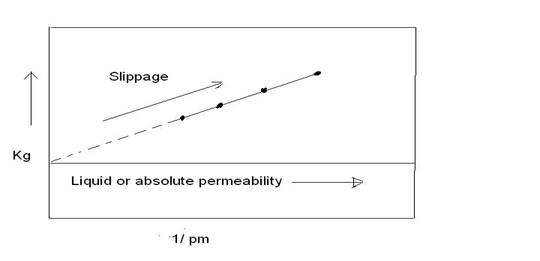# Assignment Help With The Klinkenberg Effect

Klinkenberg (1941) discovered that permeability measurements made with air as the flowing fluid showed different results from permeability measurements made with a liquid as the flowing fluid. The permeability of a core sample measured by flowing air is always greater than the permeability obtained when a liquid is the flowing fluid. Klinkenberg postulated, on the basis of his laboratory experiments, that liquids had a zero velocity at the sand grain surface, while gases exhibited some finite velocity at the sand grain surface. In other words, the gases exhibited slippage at the sand grain surface. This slippage resulted in a higher flow rate for the gas at a given pressure differential. Klinkenberg also found that for a given porous medium as the mean pressure increased the calculated permeability decreased.Mean pressure is defined as upstream flowing plus downstream flowing pressure divided by two, [pm = (p1 + p2)/2]. If a plot of measured permeability versus 1/pm were extrapolated to the point where 1/pm = 0, in other words, where pm = infinity, this permeability would be approximately equal to the liquid permeability. A graph of this nature is shown in Figure 2. The absolute permeability is determined by extrapolation as shown in Figure 2.The resulting straight-line relationship can be expressed as

Kg = Kl + c [ 1/pm]

Where kg = measured gas permeability

Pm = mean pressure

kL = equivalent liquid permeability, i.e., absolute permeability, k

c = slope of the lineFig. The Klinkenberg effect in gas permeability measurements